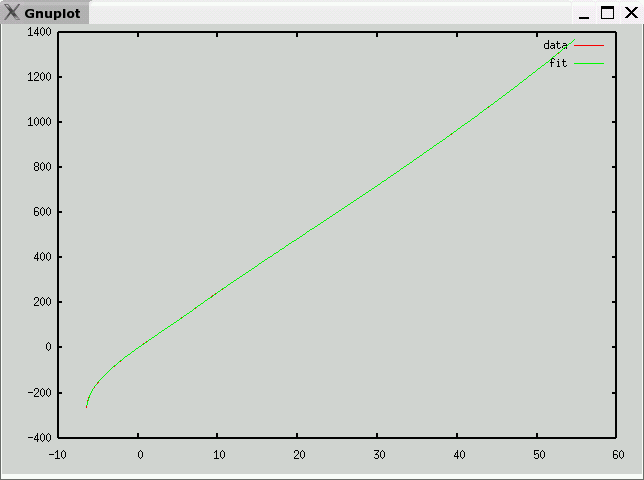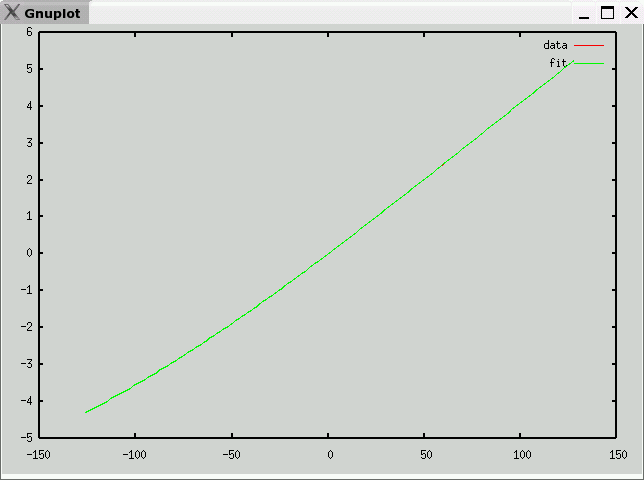# Type K Thermocouple Data and Polynomial

• Description
Ni-Cr / Ni-Al
• Data source
National Institute of Science and Technology (NIST)
• Raw Data
• Octave format
• Curve fit
• mV -> temperature (C)
e is maximum error (in degrees C)
v1 andf v2 partition the domain into three segments, each with a polynomial fit
c is vector of coefficients: a10, a9, ... a0
Note: Each column of c corresponds to one range (<v1,v1 to v2, >v2)

`e = 0.34668v1 = 11.176v2 = 22.691c =    -2.4608e-07   -2.1453e-09   1.2296e-13    6.8153e-06    3.3858e-07  -4.9588e-11   -4.2163e-05   -2.3642e-05   8.7399e-09   -3.2201e-04    9.5925e-04  -8.9155e-07    3.5591e-03   -2.4958e-02   5.8569e-05    2.8323e-03    4.3295e-01  -2.5980e-03   -9.0658e-02   -5.0349e+00   7.8935e-02    1.2429e-01    3.8316e+01  -1.6195e+00    1.9257e-01   -1.7907e+02   2.1387e+01    2.4940e+01    4.7066e+02  -1.3999e+02   -7.2259e-01   -4.0375e+02   5.6264e+02 `• temperature -> mV (range -127C-127C)
e is maximum error (in mV)
c is vector of coefficients: a10, a9, ... a0

`e = 0.00067432c =   -6.7683e-23    4.5979e-21    3.0278e-18   -1.8030e-16   -5.0020e-14    2.5828e-12    3.6705e-10   -1.3166e-07    2.6120e-05    3.9443e-02   -1.6551e-04`Previous page: Type J
Next page: Type N